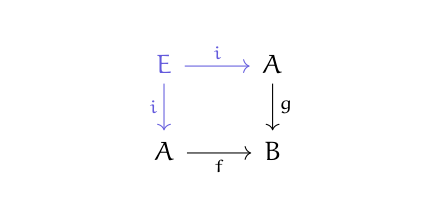# Pullbacks

A pullback is a limit over a diagram of the following shape.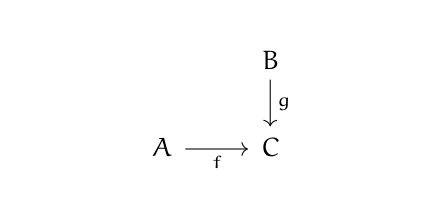The pullback is a sort of product of A and B that depends on C, and so it is sometimes written as a product with a subscript C on the product symbol: A ×C B.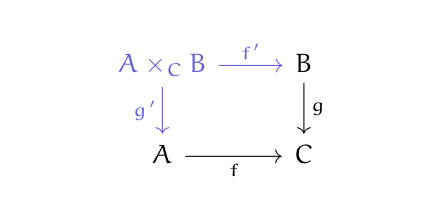In all these diagrams, the givens will be in black and the pullback will be in royal blue.

The limit of a diagram should have morphisms to all the objects in the diagram, so there should be a morphism from the pullback to C. But that morphism is redundant: if the square commutes, then the morphism on the diagonal is determined by the mormphisms on the side.

In the previous diagram, f‘ is called the pullback of f along g, and g‘ is called the pullback of g along f.

Products, intersections, inverse images, kernels, and equalizers are all pullbacks. We’ll go into each below.

The product of two objects is the pullback of the diagram mapping both objects to a terminal object 1. So pullbacks are not just analogous to products, they are a generalization of products.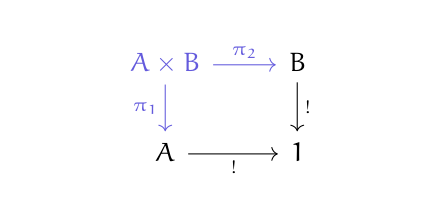The intersection of two sets U and V is the pullback of the diagram of U and V inserting into their union. So you could also think of a pullback as a generalization of intersection.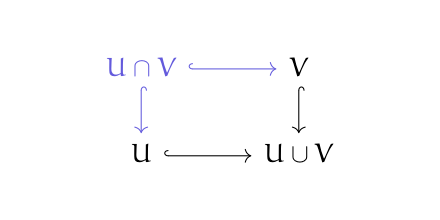Hooks on arrows indicate inclusion maps.

Let f be a function from a set A to a set B, and let C be a subset of B. Then the inverse image of C is a pullback. The function f* is the restriction of f to the inverse image of C.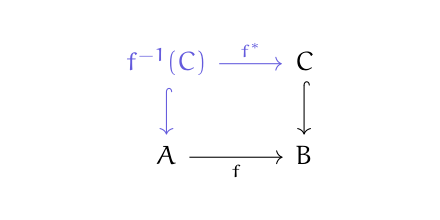Let f be a monoid homomorphism f: MN and let O be the one-element monoind. Then K, the kernel of f, is a pullback.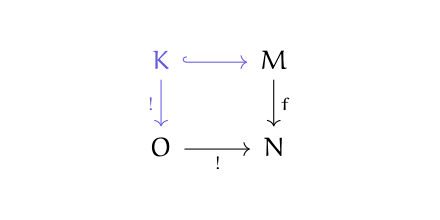The one-element monoid O is both initial and terminal, so there’s a unique map from K to O and from O into N.

Finally, equalizers are pullbacks. Given two morphisms from A into B, their pullback is an equalizer.